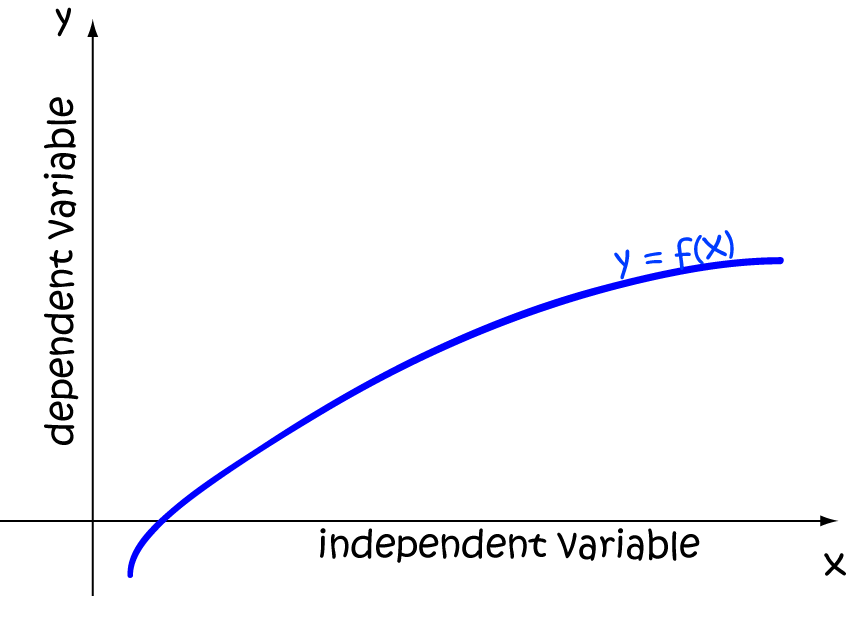# Definition of Dependent VariableA dependent variable is the output value of a function. Its value depends on the values of the input variables.

For example, in the function $y = x^3 + 3x + 4$,

• $x$ is the independent variable.
• $y$ is the dependent variable.

In the function, $V = \dfrac{1}{2}\;abh$,

• $a$ is an independent variable.
• $b$ is an independent variable.
• $h$ is an independent variable.
• $V$ is the dependent variable.

### Description

The aim of this dictionary is to provide definitions to common mathematical terms. Students learn a new math skill every week at school, sometimes just before they start a new skill, if they want to look at what a specific term means, this is where this dictionary will become handy and a go-to guide for a studen

### Audience

Year 1 to Year 12 students

### Learning Objectives

Learn common math terms starting with letter D

Author: Subject Coach
You must be logged in as Student to ask a Question.